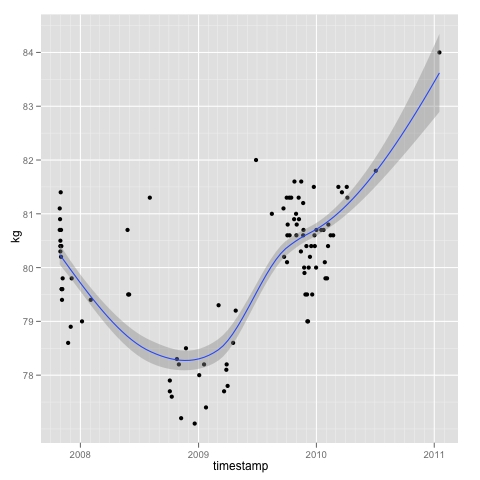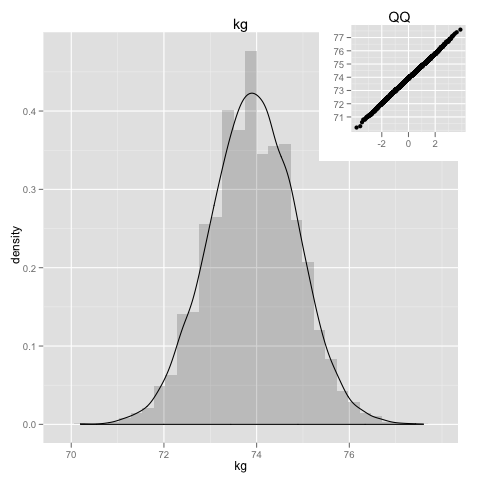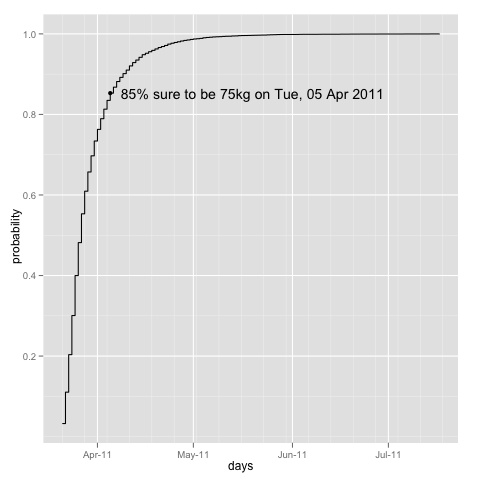[This article was first published on al3xandr3, and kindly contributed to R-bloggers]. (You can report issue about the content on this page here)
Want to share your content on R-bloggers? click here if you have a blog, or here if you don't.

Got for 2010 Xmas a very cool book called the “4 Hour Body”(thanks Jose Santos) written by Tim Ferriss who write a previous favorite of mine about productivity, the 4 hour work week.

Its an interesting book, because it has a scientific approach, it doesn’t just say do this do that and you’ll be healthy, it actually says: I(Tim Ferriss) have tried this, exactly with these steps, during this time, this is how i measured, these are the results i got and by looking at most up-to-date medical research this is the most likely explanation for these results… Notice the similar principles of AB testing.

This book couldn’t have arrived in a better time as i just peeked my heaviest weight in a long time, blame it on [insert favorite reason]… so, long story short and I am now on the 3rd week of the low-carb diet described in the book.

But of course, like with all diets, I’m quickly growing impatient of when i’m going to reach my goal (of adequate BMI), so lets use R and monte carlo simulations to generate predictions and understand better what to expect.

## Data

Have been tracking my weight using google spreadsheets, so i can get the data into R like so:

```# load the data

# remove NA's
mydata = na.omit(mydata)

# Create a new column with the proper date format
mydata\$timestamp = as.Date(mydata\$timestamp, format='%d/%m/%Y')

tail(mydata, 5)

timestamp   kg delta       day
142 2011-03-06 77.2   0.3    Sunday
143 2011-03-07 77.3   0.1    Monday
144 2011-03-08 76.9  -0.4   Tuesday
145 2011-03-09 76.4  -0.5 Wednesday
146 2011-03-11 76.1  -0.3    Friday
```

### Normality

Normality is not really a requirement for this simulation, monte carlo methods are independent of the distribution, but lets have a look of how the weight is distributed for the past 3 years.

```# include ggplot2
library(ggplot2)

gghist = function (mydata, mycolname) {
pl = ggplot(data = mydata)
subvp = viewport(width=0.35, height=0.35, x=0.84, y=0.84)

his = pl +
geom_histogram(aes_string(x=mycolname,y="..density.."),alpha=0.2) +
geom_density(aes_string(x=mycolname)) +
opts(title = names(mydata[mycolname]))

qqp = pl +
geom_point(aes_string(sample=mycolname), stat="qq") + labs(x=NULL, y=NULL) +
opts(title = "QQ")

print(his)
print(qqp, vp = subvp)
}

gghist(mydata, "kg")
```Its close to normal distribution and says my weight has been mostly(in average) 80.5kg.

## Predicting the Future

Now using Monte Carlo methods lets simulate the future based on the weight changes that happened since start of diet.

Because its going to use weight changes by day we need to do some trickery to fill in the missing days and calculate the changes for every single day. The idea for filling in missing days is; if we have only day1=81 and day3=80, then we calculate that day2=80.5, because in 2 days we see a diference of 1, then per day is 0.5.

We can confirm(prove) that this calculated assumption is a good one, by later comparing the simulation on data with all days filled in against the same data with some removed days in the middle, and confirm that results are the same.

So lets get the weight(kg) change(delta), for every day:

```library(zoo) # for missing values interpolation

fill.all.days = function (mydata, timecolname, valuecolname) {
dtrange = range(mydata[,timecolname])

# create a data frame with every single day
alldays = data.frame(tmp=seq(as.Date(dtrange), as.Date(dtrange), "days"))
colnames(alldays) = c(timecolname) # rename tmp to proper timecolname

# add the existing values
alldays = merge(alldays, mydata, by=timecolname, all=TRUE)

# fill in the missing ones
alldays[,valuecolname] = na.approx(alldays[,valuecolname])
return(alldays)
}

# from start of diet
dietdata = subset(mydata, timestamp >= "2011-01-17")
lastweight = tail(dietdata\$kg, n=1)

# fill in missing days
dietalldays = fill.all.days(dietdata, "timestamp", "kg")

# get difference day by day into data frame
kgdelta = diff(dietalldays\$kg)
dietalldays\$delta = c(0, kgdelta)

# print only the 10 last values
tail(dietalldays, 5)

timestamp    kg delta       day
50 2011-03-07 77.30  0.10    Monday
51 2011-03-08 76.90 -0.40   Tuesday
52 2011-03-09 76.40 -0.50 Wednesday
53 2011-03-10 76.25 -0.15      <NA>
54 2011-03-11 76.10 -0.15    Friday
```

### So what is going to be my weight in a week?

```predict.weight.in.days = function(days, inicialweight, deltavector) {
weight = inicialweight
for (i in 1:days) {
weight = weight + sample(deltavector, 1, replace=TRUE)
}
return(weight)
}

# simulate it 10k times
mcWeightWeek = replicate(10000, predict.weight.in.days(7, lastweight, kgdelta))

summary(mcWeightWeek)

Min. 1st Qu.  Median    Mean 3rd Qu.    Max.
70.80   74.35   75.05   75.05   75.75   78.80
```

Another good thing about monte carlo methods is that they give a distribution of the prediction, so its possible to get a feeling of how certain the average is; either very certain with a big central peak, or not that certain when the graph is flatter and all over the place:

```gghist(data.frame(kg=mcWeightWeek), "kg")
```### And when am i getting to 75kg?

```days.to.weight = function(weight, inicialweight, deltavector) {
target = inicialweight
days = 0
while (target > weight) {
target = target + sample(deltavector, 1, replace=TRUE)
days = days + 1
if (days >= 1095) # if value too crazy just interrupt the loop
break
}
return(days)
}

# simulate it 10k times
mcDays75 = replicate(10000, days.to.weight(75, lastweight, kgdelta))

summary(mcDays75)

Min. 1st Qu.  Median    Mean 3rd Qu.    Max.
2.000   4.000   7.000   9.073  11.000  82.000
```

And the cumulative distribution:

```# add dates to it, from today's date + #days
days75 = sort(Sys.Date() + mcDays75)

# get the ecdf values into a dataframe
days75.ecdf = summarize(data.frame(days=days75), days = unique(days),
ecdf = ecdf(days)(unique(days)))

# date where its 85% sure i'll reach goal

# plot
ggplot(days75.ecdf, aes(days, ecdf)) + geom_step() +
ylab("probability") +
geom_point(aes(x = prob85\$days, y = prob85\$ecdf)) +
geom_text(aes(x = prob85\$days, y = prob85\$ecdf,
label = paste("85% sure to be 75kg on",
format(prob85\$days, "%a, %d %b %Y"))),
hjust=-0.04)
```Also note that, weight loss is faster at the beginning of a diet, it tends to slow down over time, so to keep the predictions valid we need to continue record the weight and re-run the predictions frequently.

But as you see the slow carb diet seems to work, even without exercise. Tim’s book is great, focusing on the smallest things possible for the bigger results(=efficiency).

### References

Hard drive occupation prediction with R: part 1 and part 2, and thanks to Leandro Penz on the feedback.